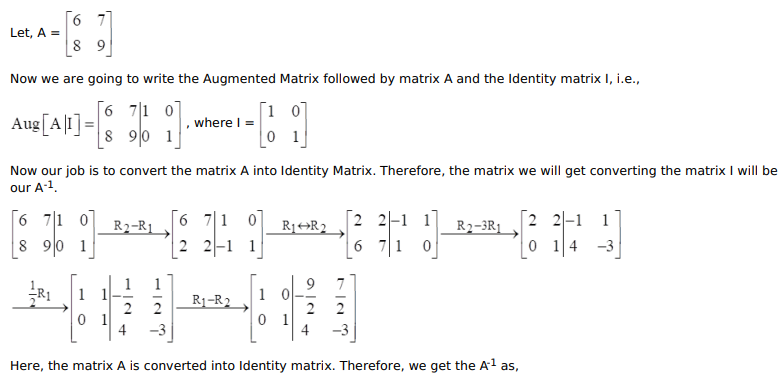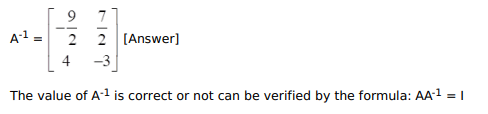# Using elementary row transformations, find the inverse of each of the following matrices:Question:

Using elementary row transformations, find the inverse of each of the following matrices:

$\left[\begin{array}{ll}6 & 7 \\ 8 & 9\end{array}\right]$

Solution: